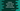# Three different Python examples to remove items from a list while iterating## How to remove items from a list while iteratng through the list:

In this post, we will learn how to remove items from a python list while iterating through it. i.e. we are iterating and also removing items simultaneously. For removing items, list.remove() method is used. For example:

``````given_list = [1,2,3,4,5]
given_list.remove(4)

print(given_list)``````

If you run this program, it will print the below output:

``[1, 2, 3, 5]``

remove() removes the first element that it finds.

### Using a loop to iterate and remove items from a list:

Let’s use one for-in loop and try to remove all elements smaller than 4 using remove :

``````given_list = [1,2,3,4,4,5,6]

for item in given_list:
if item < 4:
given_list.remove(item)

print(given_list)``````

It will print:

``[2, 4, 4, 5, 6]``

The problem is that the iterator doesn’t know if an item is removed from the list. For this example, for the first element 1, remove is called to remove it and makes 2 as the first element. But the iterator doesn’t know it and moves to the next element i.e. 3. It removes 3 and moves forward.

### Solution 1: Make a copy of the list:

We can solve this in a couple of ways. The first way is to make a copy of the list, iterate on the newly created list and call remove on the original list.

``````given_list = [1,2,3,4,4,5,6]
new_list = list(given_list)

for item in new_list:
if item < 4:
given_list.remove(item)

print(given_list)``````

It will print:

``[4, 4, 5, 6]``

This time it worked. Because, we are iterating on a list which is never modified. The drawback of this method is that we need to create one new list and for a large list, this is not a space efficient solution.

### Solution 2: Using filter():

filter() method can be used to filter out items from a list. It takes two arguments. The first one is a lambda function and the second one is the list. This lambda function is applied on each member of the list and it creates one different list. filter returns the list that is created newly.

If I write this in Python, it looks as like below:

``````given_list = [1,2,3,4,4,5,6]
new_list = list(filter(lambda num: num > 3, given_list))

print(new_list)``````

In this program, we are filtering out all the numbers which are greater than 3. If you run this program, it will print the below output:

``[4, 4, 5, 6]``

Same as the above one. But we are not modifying the original list. filter() creates one new list and we are assigning it to the variable.

### Solution 3: Using list comprehension:

We can also use list comprehension. Using list comprehension, we can iterate through the list, remove elements and then assign the newly created list to a variable.

``````given_list = [1,2,3,4,4,5,6]
new_list = [num for num in given_list if num > 3]

print(new_list)``````

It gives the same output. Similar to the above example, we are creating a new list with this method.